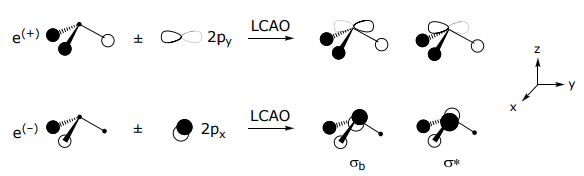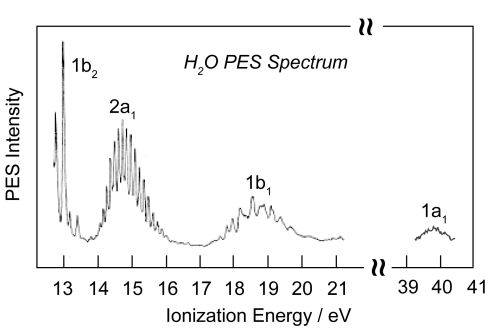# 1.11: Frontier molecular orbitals of σ-donor, π-donor and π-acceptor ligands

$$\newcommand{\vecs}{\overset { \rightharpoonup} {\mathbf{#1}} }$$ $$\newcommand{\vecd}{\overset{-\!-\!\rightharpoonup}{\vphantom{a}\smash {#1}}}$$$$\newcommand{\id}{\mathrm{id}}$$ $$\newcommand{\Span}{\mathrm{span}}$$ $$\newcommand{\kernel}{\mathrm{null}\,}$$ $$\newcommand{\range}{\mathrm{range}\,}$$ $$\newcommand{\RealPart}{\mathrm{Re}}$$ $$\newcommand{\ImaginaryPart}{\mathrm{Im}}$$ $$\newcommand{\Argument}{\mathrm{Arg}}$$ $$\newcommand{\norm}{\| #1 \|}$$ $$\newcommand{\inner}{\langle #1, #2 \rangle}$$ $$\newcommand{\Span}{\mathrm{span}}$$ $$\newcommand{\id}{\mathrm{id}}$$ $$\newcommand{\Span}{\mathrm{span}}$$ $$\newcommand{\kernel}{\mathrm{null}\,}$$ $$\newcommand{\range}{\mathrm{range}\,}$$ $$\newcommand{\RealPart}{\mathrm{Re}}$$ $$\newcommand{\ImaginaryPart}{\mathrm{Im}}$$ $$\newcommand{\Argument}{\mathrm{Arg}}$$ $$\newcommand{\norm}{\| #1 \|}$$ $$\newcommand{\inner}{\langle #1, #2 \rangle}$$ $$\newcommand{\Span}{\mathrm{span}}$$$$\newcommand{\AA}{\unicode[.8,0]{x212B}}$$

Before tackling the business of the complex, the nature of the ligand frontier orbitals must be considered. There are three general classes of ligands, as defined by their frontier orbitals: σ-donor ligands, π-donor ligands and π-acceptor ligands.

σ-donor ligands

These ligands donate two e– s from an orbital of σ-symmetry:

H(1s2 ), NH3 (2a1 lp), PR3 (2a1 lp), CH3- (2a1 lp), OH2 (b1 lp)

Note, some of these ligands are atomic, while others are LCAO-MOs. The frontier orbitals for bonding to the metal are thus are either atomic or molecular orbitals, depending on the nature of the ligand.

As an example of a molecular ligand, consider the ammonia ligand. Ammonia is formed from the LCAO between the valence orbitals of a central nitrogen and the three 1s orbitals of three hydrogens,To begin this problem, the symmetry-adapted linear combinations of the three 1s orbitals must be determined. Hence, the basis will be derived from the H orbitals. Because the H(1s) orbitals can only form σ bonds, the choice of 3 σ N–H bonds is an appropriate basis set,The transformation properties of the σ bonds (in C3v symmetry) are as follows:

$E \begin{bmatrix} σ_{1} \\ σ_{2} \\ σ_{3} \end{bmatrix} \rightarrow \begin{bmatrix} σ_{1} \\ σ_{2} \\ σ_{3} \end{bmatrix} \rightarrow \begin{bmatrix} 0 & 1 & 0 \\ 0 & 0 & 1 \\ 1 & 0 & 0 \end{bmatrix}$

$C_{3} \begin{bmatrix} σ_{1} \\ σ_{2} \\ σ_{3} \end{bmatrix} \rightarrow \begin{bmatrix} σ_{1} \\ σ_{2} \\ σ_{3} \end{bmatrix} \rightarrow \begin{bmatrix} 1 & 0 & 0 \\ 0 & 1 & 0 \\ 0 & 0 & 1 \end{bmatrix}$

$σ_{v} \begin{bmatrix} σ_{1} \\ σ_{2} \\ σ_{3} \end{bmatrix} \rightarrow \begin{bmatrix} σ_{1} \\ σ_{2} \\ σ_{3} \end{bmatrix} \rightarrow \begin{bmatrix} 1 & 0 & 0 \\ 0 & 0 & 1 \\ 0 & 1 & 0 \end{bmatrix}$

These representation of this basis may be quickly ascertained by realizing that only bonds (or H(1s) orbitals) that do not move will contribute to the trace of the matrix representation,

$\begin{array}{c|ccc} \mathrm{C}_{3 \mathrm{v}} & \mathrm{E} & 2 \mathrm{C}_{3} & 3 \sigma_{\mathrm{v}} \\ \hline \Gamma_{\sigma} & 3 & 0 & 1 \end{array}\rightarrow a_{1} + e$

Projecting out the a1 and e SALCs of the 3H orbitals, we realize that the transformation properties of the H orbitals are preserved in the C3 rotational subgroup,Application of the projection operator in the cyclic C3 point group is an easy task – can simply read out the projections,

\begin{aligned}
&\mathrm{P}^{\mathrm{a}_{1}}\left(\sigma_{1}\right) \rightarrow 1 \cdot \mathrm{E} \sigma_{1}+1 \cdot \mathrm{C}_{3} \sigma_{1}+1 \cdot \mathrm{C}_{3}^{2} \sigma_{1}=\sigma_{1}+\sigma_{2}+\sigma_{3} \\
&\mathrm{P}^{\mathrm{e}(1)}\left(\sigma_{1}\right) \rightarrow 1 \cdot \mathrm{E} \sigma_{1}+\left(\varepsilon^{*}\right) \mathrm{C}_{3} \sigma_{1}+\varepsilon \mathrm{C}_{3}^{2} \sigma_{1}=\sigma_{1}+\varepsilon^{*} \sigma_{2}+\varepsilon \sigma_{3} \\
&\mathrm{P}^{\mathrm{e}(2)}\left(\sigma_{1}\right) \rightarrow 1 \cdot \mathrm{E} \sigma_{1}+\varepsilon \mathrm{C}_{3} \sigma_{1}+\left(\varepsilon^{*}\right) \mathrm{C}_{3}^{2} \sigma_{1}=\sigma_{1}+\varepsilon \sigma_{2}+\varepsilon^{*} \sigma_{3}
\end{aligned}

Taking appropriate linear combinations and normalizing,Only orbitals of the same symmetry can form a LCAO; thus the a1 SALC of the 3H(1s) orbitals can only combine with the a1 orbitals of the central N (i.e., the 2s and 2pz valence orbitals),he 2py orbital combines with e(+) , it is orthogonal to e(–), whereas the opposite is true for 2px orbital,The MO is constructed by overlapping orbitals of the same symmetry. The greater the overlap, the greater the splitting between the orbitals. Note that the a1 SALC participates in two types of σ interactions, one with the 2s orbital and one with the 2pz orbital of nitrogen. The highest energy orbital, the ligand HOMO orbital, is used for bonding to the metal. This orbital too is composed of two types of interactions: (i) it is Lσ(a1)–N(2s) antibonding and (ii) Lσ(a1)–N(2pz) bonding in character. The energy of the atomic orbitals are shown in parenthesis.Simple hybridization arguments predict two different of bond energies for NH3: (1) the lone pair and (2) the σ N–H bond. The MO diagram on the other hand predicts three different energies. The photoelectron spectrum of NH3 exhibits three ionization energies, thus verifying the MO bonding model.A second molecular ligand is water. The ligand has two lone pairs, but only one is used in bonding to the metal. The reason for this electronic asymmetry and why only one lone pair is only available for bonding becomes evident from the electronic structure of the water molecule.The basis set for water is the 2H(1s) orbitals and the O(2s, 2pz, 2px, 2py) atomic orbitals. The proper symmetry adapted linear combination for the 2H(1s) orbitals may be ascertained using the above σ bonds.

\begin{aligned}
&\begin{array}{c|cccc}
\mathbf{C}_{\mathbf{2 v}} & \mathrm{E} & \mathrm{C}_{2} & \sigma_{\mathrm{v}}(\mathrm{xz}) & \sigma_{\mathrm{v}}(\mathrm{yz}) \\
\hline \Gamma_{\sigma} & 2 & 0 & 2 & 0 & \rightarrow a_{1} + b_{1} \\
\hline \sigma_{1} \rightarrow & \sigma_{1} & \sigma_{2} & \sigma_{1} & \sigma_{2}
\end{array}\\
\end{aligned}

Applying the projection operator,

\begin{aligned}
&\mathrm{P}^{\mathrm{a}_{1}}\left(\sigma_{1}\right) \rightarrow 1 \cdot \overbrace{ {\mathrm{E} \sigma_{1}}}^{ \sigma_{1}}+1 \cdot \overbrace{ {\mathrm{C}_{2} \sigma_{1}} }^{\sigma_{2}}+1 \cdot \overbrace{ {\sigma(\mathrm{xz}) \cdot \sigma_{1}} }^{\sigma_{1}}+1 \cdot \overbrace{ {\sigma(\mathrm{yz}) \cdot \sigma_{1}} }^{\sigma_{2}} \rightarrow \psi_{\mathrm{a}_{1}}=\frac{1}{\sqrt{2}}\left(\sigma_{1}+\sigma_{2}\right)\\
&\mathrm{P}^{\mathrm{b}_{1}}\left(\sigma_{1}\right) \rightarrow 1 \cdot \mathrm{E} \sigma_{1}+(-1) \cdot \mathrm{C}_{2} \sigma_{1}+1 \cdot \sigma(\mathrm{xz}) \cdot \sigma_{1}+(-1) \cdot \sigma(\mathrm{yz}) \cdot \sigma_{1} \rightarrow \psi_{\mathrm{b}}=\frac{1}{\sqrt{2}}\left(\sigma_{1}-\sigma_{2}\right)
\end{aligned}

The s and pz orbitals on O have a1 symmetry and thus will mix with the Lσ(a1), the px has b1 symmetry and will mix with Lσ(b1) and the py orbital is rigorously nonbonding, i.e., does not have a symmetry counterpart of the O atom, and hence no LCAO is formed using this orbital.Again, the simple hybridization picture of bonding is shown to be incorrect, and the MO bonding model is corroborated.π-donors

π-donors In addition to donating electron density to a metal via a σ-bond, es may be provided to the metal via a π-symmetry interaction. π-donor ligands include X(halide), amide (NR2 ), sulfide (S2–), oxide (O2–), alkoxide (RO ).The amide MO may be constructed by beginning with the MO of “planar” NH3, followed by its perturbation upon removal of H+,π-acceptors

This class of ligands donate es from a σ orbital and they accept es from the metal into an empty π* orbital. CO is the archetype of this ligand class. Other π-acceptors are NO+, CN, CNR. Consider the MO diagram of CO below; the HOMO is filled and of σ-symmetry, the LUMO is empty and of π* symmetry.This page titled 1.11: Frontier molecular orbitals of σ-donor, π-donor and π-acceptor ligands is shared under a CC BY-NC-SA 4.0 license and was authored, remixed, and/or curated by Daniel Nocera via source content that was edited to the style and standards of the LibreTexts platform; a detailed edit history is available upon request.